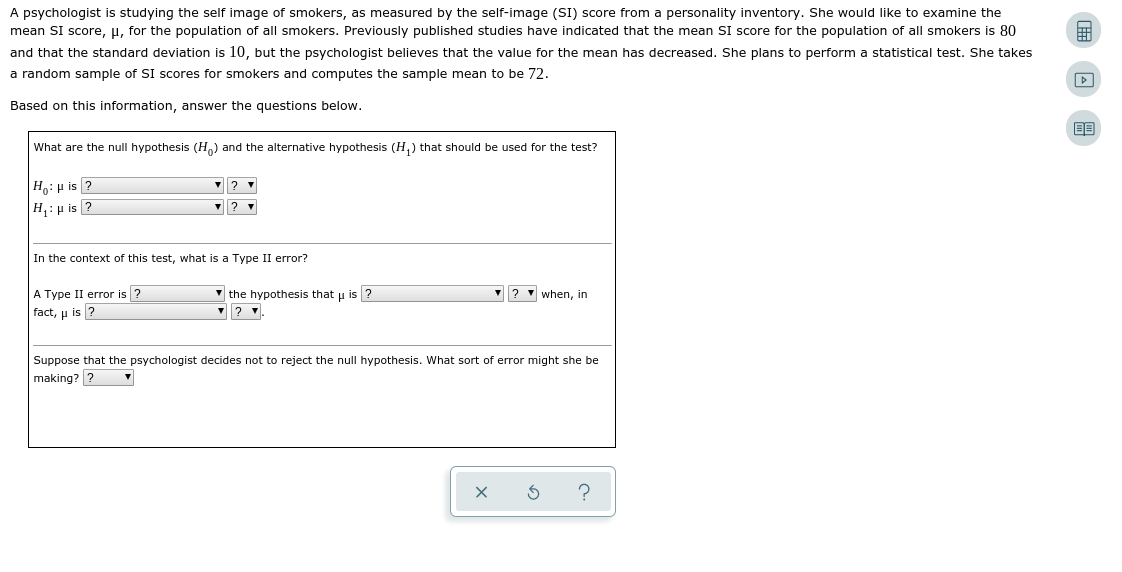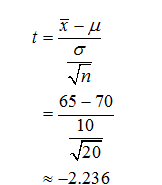# A psychologist is studying the self image of smokers, as measured by the self-image (SI) score from a personality inventory. She would like to examine themean SI score, µ, for the population of all smokers. Previously published studies have indicated that the mean SI score for the population of all smokers is 80and that the standard deviation is 10, but the psychologist believes that the value for the mean has decreased. She plans to perform a statistical test. She takesa random sample of SI scores for smokers and computes the sample mean to be 72.Based on this information, answer the questions below.What are the null hypothesis (H) and the alternative hypothesis (H,) that should be used for the test?H: µ is ?H: p is ?In the context of this test, what is a Type II error?A Type II error is ?fact, p is ?v the hypothesis that u is ?when, inSuppose that the psychologist decides not to reject the null hypothesis. What sort of error might she bemaking? ?

Question
60 views

An electronics manufacturing process has a scheduled mean completion time of

70

minutes. It is claimed that, under new management, the mean completion time,

μ

, is less than

70

minutes. To test this claim, a random sample of

20

completion times under new management was taken.

The sample had a mean completion time of

65

minutes and a standard deviation of

10

minutes. Assume that the population of completion times under new management is normally distributed. At the

0.05

level of significance, can it be concluded that the mean completion time,

μ

, under new management is less than the scheduled mean?

Perform a one-tailed test. Then fill in the table below.

Carry your intermediate computations to at least three decimal places and round your answers as specified in the table.help_outlineImage TranscriptioncloseA psychologist is studying the self image of smokers, as measured by the self-image (SI) score from a personality inventory. She would like to examine the mean SI score, µ, for the population of all smokers. Previously published studies have indicated that the mean SI score for the population of all smokers is 80 and that the standard deviation is 10, but the psychologist believes that the value for the mean has decreased. She plans to perform a statistical test. She takes a random sample of SI scores for smokers and computes the sample mean to be 72. Based on this information, answer the questions below. What are the null hypothesis (H) and the alternative hypothesis (H,) that should be used for the test? H: µ is ? H: p is ? In the context of this test, what is a Type II error? A Type II error is ? fact, p is ? v the hypothesis that u is ? when, in Suppose that the psychologist decides not to reject the null hypothesis. What sort of error might she be making? ? fullscreen
check_circle

star
star
star
star
star
1 Rating
Step 1

Hey, since there are multiple questions posted, we will answer first question. If you want any specific question to be answered then please submit that question only or specify the question number in your message.

Step 2

Null hypothesis:

µ=70.

Alternative hypothesis:

µ<70.

This is a left tailed test.

Since the population standard deviation is unknown, the appropriate test is one sample t-test.

Here, the sample mean, x-bar is 65.

Population mean, µ is 70.

Sample standard deviation, s = 10.

Sample size, n is 20.

The test statistic value can be obtained as follows:...

### Want to see the full answer?

See Solution

#### Want to see this answer and more?

Solutions are written by subject experts who are available 24/7. Questions are typically answered within 1 hour.*

See Solution
*Response times may vary by subject and question.
Tagged in